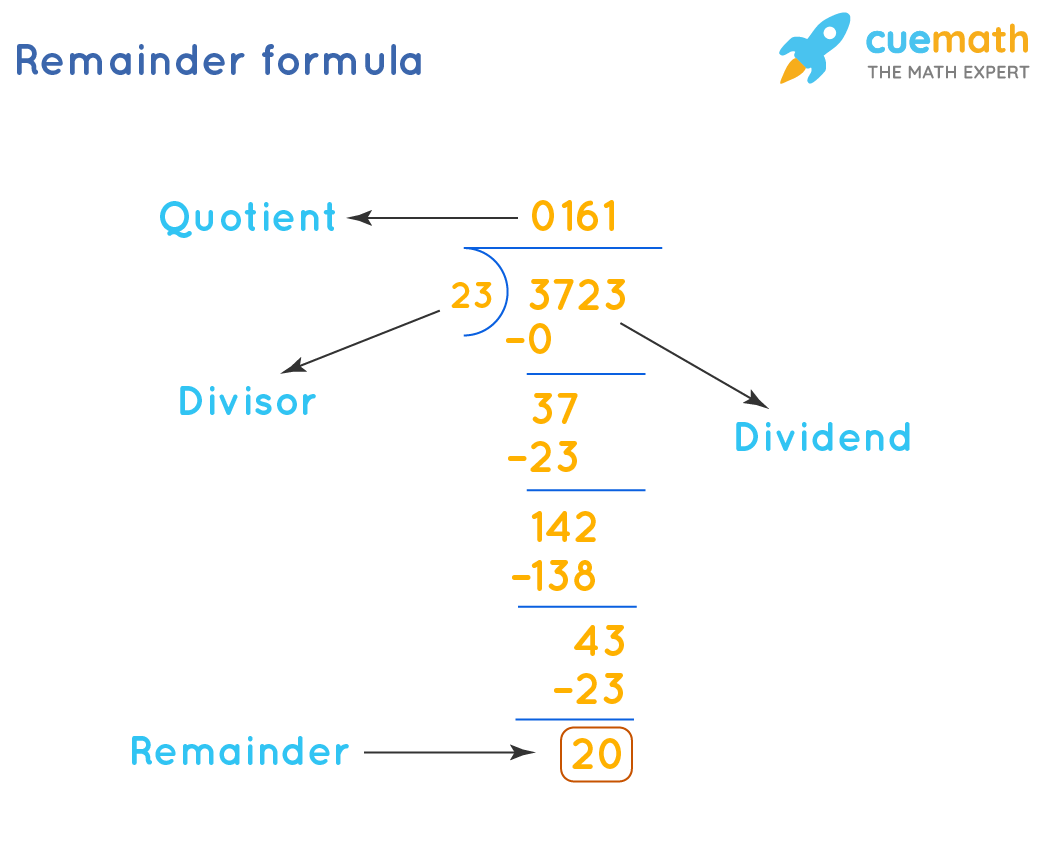# Remainder Formula

Go back to  'Math Formulas'

The remainder is the number or value that is left when the dividend is not completely divisible by the divisor. The remainder formula helps in the calculation of the remainder obtained after the division operation between any two values. The division operation in terms of operands can be represented as:

Dividend = Divisor x Quotient + Remainder

## What is the Remainder Formula?

The general formula to calculate the remainder is given as:## Solved Examples Using Remainder Formula

### Check if the answer you got is correct.

Solution:

To find: Remainder

Given: Dividend = 3723 and Divisor = 23

By long division:Hence, Reaminder = 20

Verification:

Using Remainder Formula,

Dividend = Divisor × Quotient + Remainder

3723 = 23 × 161 + 20

3723 = 3703 + 20

3723 = 3723

Since we have the same values on both the sides, our answer is correct.

### A number when divided by 25 leaves remainder 4 and quotient as 11. Find that number.

Solution:

To find: Dividend (number)

Given:

Divisor = 25

Quotient = 11

Remainder = 4

We know,

Dividend = Divisor × Quotient + Remainder

= 25 × 11 + 4

= 279